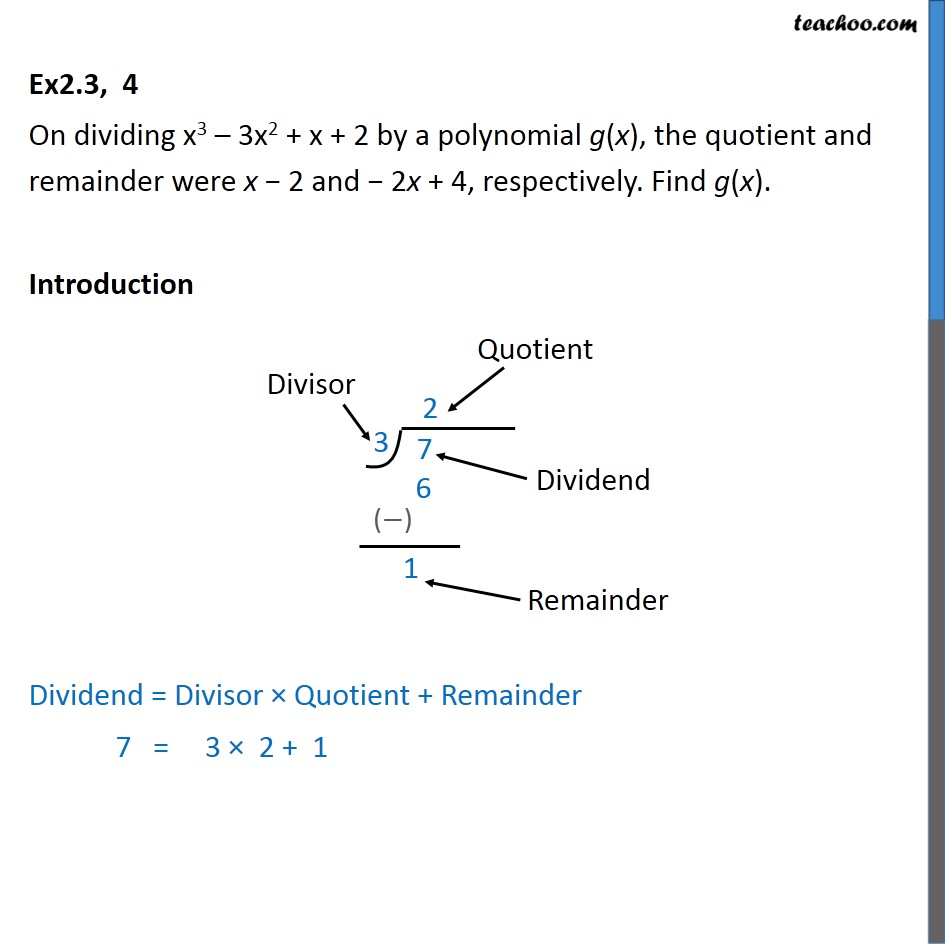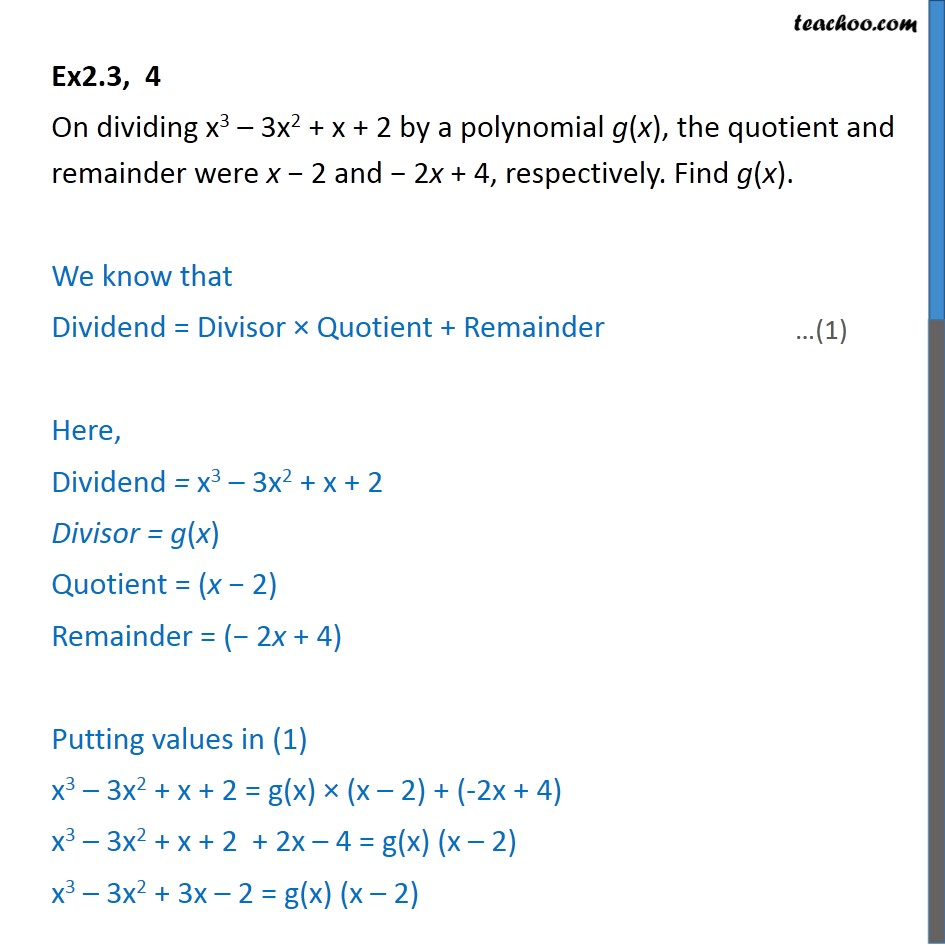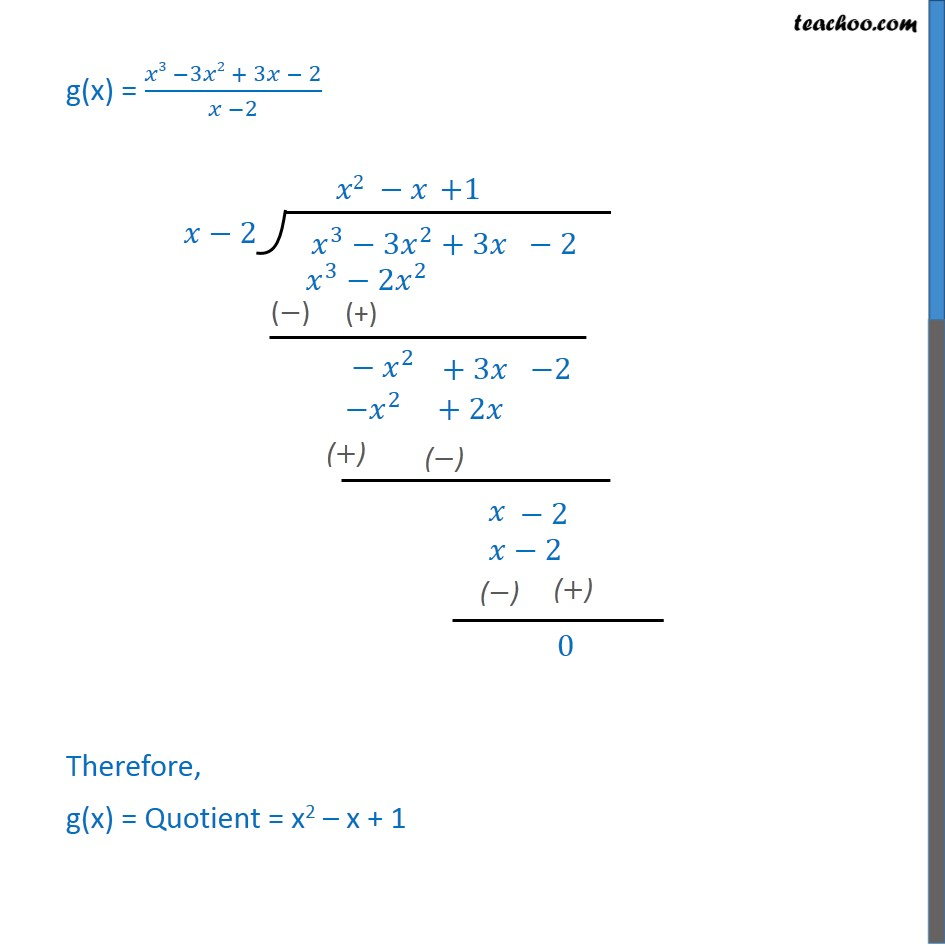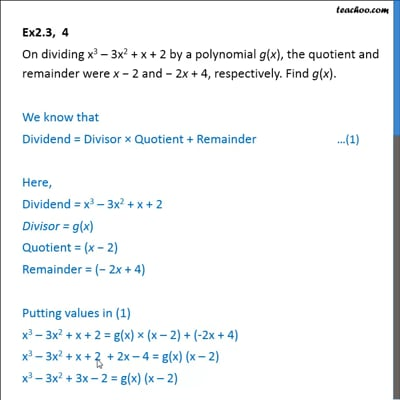Ex 2.3

Chapter 2 Class 10 Polynomials
Serial order wiseThis video is only available for Teachoo black users

Introducing your new favourite teacher - Teachoo Black, at only ₹83 per month

### Transcript

Ex2.3, 4 On dividing x3 3x2 + x + 2 by a polynomial g(x), the quotient and remainder were x 2 and 2x + 4, respectively. Find g(x). Introduction Dividend = Divisor Quotient + Remainder 7 = 3 2 + 1 Ex2.3, 4 On dividing x3 3x2 + x + 2 by a polynomial g(x), the quotient and remainder were x 2 and 2x + 4, respectively. Find g(x). We know that Dividend = Divisor Quotient + Remainder Here, Dividend = x3 3x2 + x + 2 Divisor = g(x) Quotient = (x 2) Remainder = ( 2x + 4) Putting values in (1) x3 3x2 + x + 2 = g(x) (x 2) + (-2x + 4) x3 3x2 + x + 2 + 2x 4 = g(x) (x 2) x3 3x2 + 3x 2 = g(x) (x 2) g(x) = ( 3 3 2 + 3 2)/( 2) Therefore, g(x) = Quotient = x2 x + 1